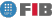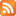# Algorithmics, Computability and Complexity (ALCC)

Credits Dept. Type Requirements
9.0 (7.2 ECTS) CS
• Compulsory for DCSYS
PS - Prerequisite for DCSFW

## Instructors

 Person in charge: (-) Others: (-)

## General goals

Upon finishing this subject, students should have an understanding of the basic elements of Theory of Computation, including Automata and Formal Language Theory, Computability Theory, Complexity Theory and Algorithmics. Students gain the understanding and the experience needed to be able to classify problems. Problems are classified according to the existence or absence of algorithms for solving them, and, in the case of the latter, based on the possibility of finding an efficient alfgorithmic solution.

## Specific goals

### Knowledges

1. Understand some abstract models of classic calculation and their scope and limitations.
2. Know how to classify problems by classes of complexity as defined by various calculation models. In particular, understanding what negative results mean (irregularity, intractability, undecidability).
3. Understand the concept of problem reduction, and its use in demonstrating whether or not a problem is tractable.
4. Learn how to analyze an algorithm"s cost and a problem"s computational complexity. Understand the qualitative difference between polynomic time and exponential time.
5. Learn the computational problems for which no polynomic algorithms are currently available.
6. Learn some of the algorithmic techniques for tackling difficult problems; in particular, complete NP problems.
7. Understanding the use of logical-mathematical notation to represent computational problems and calculation models.

### Abilities

(no available informacion)

### Competences

1. Critical, logical-mathematical reasoning.
2. Ability to understand and build logical-mathematical constructions.
3. Ability to solve problems through the application of scientific and engineering methods.
4. Ability to apply mathematical knowledge and logic in solving problems.
5. Ability to think in abstract terms. Ability to tackle new problems by consciously using strategies that have proved useful in solving previous problems.
6. Ability to act independently: Know how to work on one"s own with just the bare minimum of knowledge and guidance.
7. Ability to effectively convey one"s ideas in writing.
8. Intellectual curiosity and openness.

## Contents

Estimated time (hours):

 T P L Alt Ext. L Stu A. time Theory Problems Laboratory Other activities External Laboratory Study Additional time

1. Part I. Foundations
T      P      L      Alt    Ext. L Stu    A. time Total
12,0 12,0 0 0 0 24,0 0 48,0
Mathematical notions.
Decidability.
Complexity.

2. Part II. Algorithms
T      P      L      Alt    Ext. L Stu    A. time Total
12,0 12,0 0 0 0 24,0 0 48,0
Exhaustive search.
Greedy algorithms.
Dynamic programming.

3. Part III. Computation
T      P      L      Alt    Ext. L Stu    A. time Total
12,0 12,0 0 0 0 24,0 0 48,0
NP-completeness.
String matching.
Parsing.

4. Part IV. Classes
T      P      L      Alt    Ext. L Stu    A. time Total
1,0 11,0 0 0 0 12,0 0 24,0
Language classification.

 Total per kind T P L Alt Ext. L Stu A. time Total 37,0 47,0 0 0 0 84,0 0 168,0 Avaluation additional hours 9,0 Total work hours for student 177,0

## Docent Methodolgy

The first half of each two-hour class covers theory and the second half is spent doing exercises. Moreover, there will be a continued evaluation which will consist of three exams (for parts I-III) undertaken during class time.

## Evaluation Methodgy

The subject has a continued evaluation system and a final exam. The continued evaluation consists of 3 tests made during the semester, while the final exam contains 4 parts.
If the grades of the tests are p1, p2, p3, and the grades of the problems of the final exam are f1, f2, f3, f4, then the global grade of the subject is

max(p1,f1) + max(p2,f2) + max(p3,f3) + f4

The four values of the previous summation respectively correspond to the four parts in which the subject is divided. Parts I-III contribute with 3 points each, while part IV contributes with 1 point.

The tests will take place at usual classroom hours, shortly after finishing the topics they include. They will be announced at Racó at the beginning of the course.

## Basic Bibliography

• Michael Sipser Introduction to the theory of computation, Thomson Course Technology, 2006.
• G. Brassard, T. Bratley Fundamentos de algoritmia, Prentice Hall,, 1996.
• Maria Serna ... [et al.] Els Límits de la computació : indecidibilitat i NP-completesa, Edicions UPC, 2001.
• Thomas H. Cormen ... [et al.] Introduction to algorithms, MIT Press, 2001.

## Complementary Bibliography

• Efim Kinber, Carl Smith Theory of computing : a gentle introduction, Prentice-Hall, 2001.
• Michael R. Garey, David S. Johnson Computers and intractability : a guide to the theory of NP-Completeness, W.H. Freeman, 1979.
• Ian Parberry Problems on algorithms, Prentice Hall, 1995.
• Rafel Cases, Lluís Màrquez Llenguatges, gramàtiques i autòmats : curs bàsic, Edicions UPC, 2003.

(no available informacion)

## Previous capacities

Programming knowledge and data structures.
Familiarity with logico-mathematical reasoning.© Barcelona school of informatics - Contact -RSS
This website uses cookies to offer you the best experience and service. If you continue browsing, it is understood that you accept our cookies policy.
Classic version Mobile version